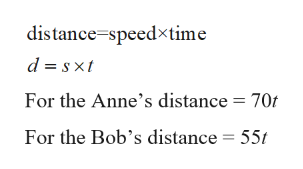# This math problem is from Pre-Calculus 1st quarter. Suppose that cities X and Y are exactly 812.5 miles apart. On a certain day, Anne leaves from city X traveling at a rate of 70 mph towards city Y. At the same time, and taking the same route, Bob leaves city Y traveling at a rate of 55 mph towards city X. How far from city Y will Anne and Bob pass each other?  a) Write an equation to help you solve this problem. You may use variables d for distance or t for time.

Question
1 views

This math problem is from Pre-Calculus 1st quarter.

Suppose that cities X and Y are exactly 812.5 miles apart. On a certain day, Anne leaves from city X traveling at a rate of 70 mph towards city Y. At the same time, and taking the same route, Bob leaves city Y traveling at a rate of 55 mph towards city X. How far from city Y will Anne and Bob pass each other?

a) Write an equation to help you solve this problem. You may use variables d for distance or t for time.

check_circle

star
star
star
star
star
1 Rating
Step 1

Since they are 812.5 miles apart and they are traveling tow...help_outlineImage Transcriptionclosedistance speedxtime d sxt For the Anne's distance = 70t For the Bob's distance = 55t fullscreen

### Want to see the full answer?

See Solution

#### Want to see this answer and more?

Solutions are written by subject experts who are available 24/7. Questions are typically answered within 1 hour.*

See Solution
*Response times may vary by subject and question.
Tagged in

### Other